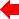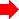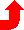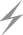Computer Aids for VLSI Design
Steven M. Rubin

Chapter 6: Dynamic Analysis Tools

This chapter was contributed by Robert W. Hon, Cadence Design Systems Inc.Section 3 of 8## 6.3 Logic-Level Simulators

Logic-level simulators replace the analog waveforms of circuit-level simulators with the three values 0, 1, and X (undefined). Circuit elements are modeled as simple switches or gates connected by wires the propagation delay of which is typically zero. Logic-level simulators provide the ability to simulate larger designs than circuit-level simulators can, at the expense of detail. Logic-level simulators may simulate at the device level or at the gate level.

### 6.3.1 Switch-Level Simulators

Switch-level simulators [Bryant] model circuits as a collection of transistors and wires, and must be provided with connectivity information. This information consists of a list of transistors and wires, which provide the equivalent of a schematic of the design. Transistors are modeled as simple switches and wires are modeled as idealized, zero-delay conductors. Usually a simple delay model is incorporated into the transistor model; for example, unit-delay in switching. Other delay information such as transistor delays derived in a previous circuit simulation, may be incorporated if available.

Processing is a matter of solving simplified equations based on approximate circuit theory. Rudimentary timing information, within the limits of the simplified model, is available for the nodes being monitored. In spite of the approximations involved, the timing information may be sufficient to determine overall system parameters such as worst-case delays and maximum clock rates.

### 6.3.2 Gate-Level Simulators

Gate-level simulators use the same logic values as switch-level simulators use, but circuit elements are modeled at the gate rather than the transistor level. Gate-level simulators can be used to simulate still larger designs in terms of logic values. However, since they are based on a model of gates, they cannot easily model some common VLSI structures (for example, pass transistors, tri-state bus structures).

Input to gate-level simulators is a schematic of the circuit in terms of logic gates (for example, NAND, OR, D-flip flop) and wires. Some delay is associated with each gate. Usually the schematic is directly available from the schematic-entry system used to draw the design. Truth tables, extended to include the undefined logic value, can be used to model each gate. Figure 6.3 shows the truth table for a two-input AND gate.
 In1 In2 Out 0 0 0 0 1 0 0 X 0 1 0 0 1 1 1 1 X X X 0 0 X 1 X X X X
FIGURE 6.3 Truth table for two-input AND gate.

Processing is similar to that in switch-level simulators, although larger designs can often be simulated because the computational elements are more complex. In addition, the lack of certain constructs available in a transistor-based model reduces the complexity of the computation involved.

### 6.3.3 Multiple-State Simulators

In order to obtain more complete information than is provided by the values 0, 1, and X, and yet retain the advantage of being able to handle complex designs, some logic-level simulators are augmented by a larger set of logic levels [VLSI]. It is not uncommon to find commercially offered simulators with a dozen or more states. States are added to provide finer resolution of conflicts at nodes; for example, when a resistive pullup is in opposition to an output that is driving the same output low or when a tri-state bus is in a high-impedance state. Component behavior is specified by large truth tables, as in the simpler three-state simulators.PreviousTable of Contents NextSteven M. Rubin Static Free Software